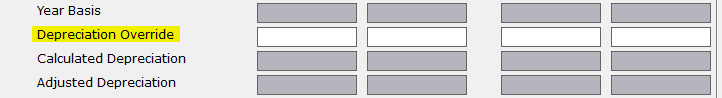# The depreciation calculation is incorrect in a tax return

You are here:
• The depreciation calculation is incorrect in a tax return

The depreciation calculation is incorrect in a tax return

SYMPTOMS

The tax preparer has a tax return where they feel the depreciation calculation is incorrect. It may have too large of a depreciation deduction.

CAUSE

When an amount has been entered for prior year depreciation, and the amount is less than the amount calculated by Simple Tax 1040, the difference is added on to the current year depreciation. The prior year depreciation amount may be incorrect due to a conversion from other tax software.

RESOLUTION

• Press CTRL+N to open the All Forms & Schedules, Depreciation screen.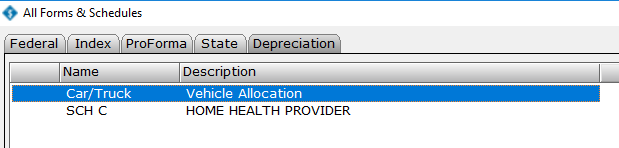• Double-click the business activity to open the Business Activity Asset window.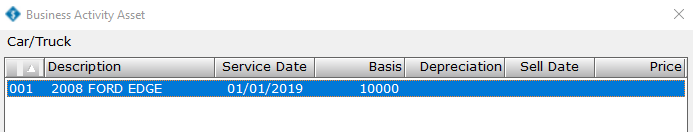• Double-click the asset that is generating too much depreciation.
• Click the Depreciation tab.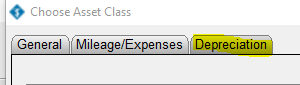• Type the correct amount of prior year depreciation in the Adjusted Depreciation box.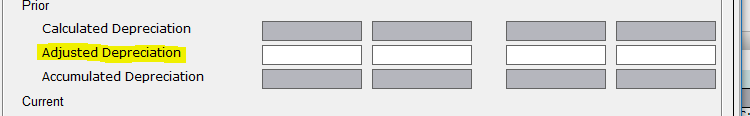• If the Adjusted Depreciation amount is still incorrect, type the correct amount of depreciation in the Depreciation Override box.